# Algebra - math word problems

1. The rod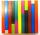The rod is painted in four colors. 55% of the bar is painted in blue, green 0.2 of rod, 1/8 is brown and the remaining 45 cm of white. How long is rod?
2. Polygon 42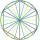Which polygon has 42 more diagonals than sides?
3. Family parcelsIn father will he divided the land so that the older son had three bigger part than younger son. Later elder son gave 2.5 ha field to younger and they had both the same. Determine the area of family parcel.
4. Tea blendTea blends are maked from two kinds of tea. In standard tea mixture are two teas in the ratio 1:3 and 40 g costs 42 CZK. In the premium tea mixture are weighing two teas in the ratio 1:1 and 50 grams costs 60 CZK. How much cost 10 grams of more expensive
5. Digits A, B, CFor the various digits A, B, C is true: the square root of the BC is equal to the A and sum B+C is equal to A. Calculate A + 2B + 3C. (BC is a two-digit number, not a product).
6. Jam cakes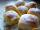Mom baked a third of plum jam cakes, one third cheesecakes and 18 poppy. How many cakes she had bake?
7. Ten dices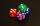When you hit ten dices at the same time you get average 35. How much do you hit if every time you get six, you're throwing the dice again?
8. RTriangle 17The hypotenuse of a right triangle is 17 cm. If you decrease both two legs by 3 cm you will reduce the hypotenuse by 4 cm. Determine the length of this legs.
9. Miraculous treeMiraculous tree grows so fast that the first day increases its height by half the total height of the second day by the third, the third day by a quarter, etc. How many times will increase its height after 6 days?
10. Tenth memberCalculate the tenth member of geometric sequence when given: a1=1/2 and q=2
11. The city 2Today lives 167000 citizens in city. How many citizens can we expect in 11 years if their annual increase is 1%?
12. Heating plant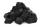Workers dump imported coke at heating plant. On the first day used half of the amount, the second day three quarters the rest and on the third day left 120 tons. How much coke they dump at heating plant?
13. Insert into GPBetween numbers 5 and 640 insert as many numbers to form geometric progression so sum of the numbers you entered will be 630. How many numbers you must insert?
14. Two cars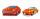From the town A to town B started two cars. The first at 7:00 at average speed 60 km per hour, the second at 10:00 at average speed 100 km per hour. The first car will not stay in B, and on the way back meet the second car at half way from A to B. At what
15. Half life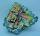Determine the half life of bismuth, when bismuth weight from the original weight of 32 g was only 2 grams in 242 minutes.
16. Rotary cylinder 2Base circumference of the rotary cylinder has same length as its height. What is the surface area of cylinder if its volume is 250 dm3?
17. Saving per cents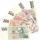The first day I save 1 cent and every next day cent more. How many I saved per year (365 days)?
18. Warehouse carsFrom the warehouse started truck at speed 40km/h. After 1hour 30mins started from the same place same direction a car at speed 70 km/h. For how long and at what distance from the warehouse overtake a truck?
19. Toys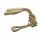The toy shop sold the same number of balls for 62 CZK and skipping ropes for 34 CZK. They income was 576 CZK. How many sold balls and how many skipping ropes?
20. Hollow sphereSteel hollow sphere floats on the water plunged into half its volume. Determine the outer radius of the sphere and wall thickness, if you know that the weight of the sphere is 0.5 kg and density of steel is 7850 kg/m3

Do you have an interesting mathematical word problem that you can't solve it? Submit math problem, and we can try to solve it.

We will send a solution to your e-mail address. Solved examples are also published here. Please enter the e-mail correctly and check whether you don't have a full mailbox.

Please do not submit problems from current active competitions such as Mathematical Olympiad, correspondence seminars etc...